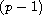Electron. J. Diff. Equ., Vol. 2011 (2011), No. 43, pp. 1-9.

### Existence and multiplicity of solutions for divergence type elliptic equations Lin Zhao, Peihao Zhao, Xiaoxia Xie

Abstract:
We establish the existence and multiplicity of weak solutions of a problem involving a uniformly convex elliptic operator in divergence form. We find one nontrivial solution by the mountain pass lemma, when the nonlinearity has a-superlinear growth at infinity, and two nontrivial solutions by minimization and mountain pass when the nonlinear term has a-sublinear growth at infinity.

Submitted January 1, 2011. Published March 31, 2011.
Math Subject Classifications: 35A15, 35J20, 35J62.
Key Words: Nonlinear elliptic equations; uniformly convex; mountain pass lemma; three critical points theorem.

Show me the PDF file (218 KB), TEX file, and other files for this article.Lin Zhao School of Mathematics and Statistics, Lanzhou University Lanzhou, Gansu 730000, China email: zhjz9332003@gmail.com Peihao Zhao School of Mathematics and Statistics, Lanzhou University Lanzhou, Gansu 730000, China email: zhaoph@lzu.edu.cn Xiaoxia Xie School of Mathematics and Statistics, Lanzhou University Lanzhou, Gansu 730000, China email: xiexx06@lzu.cn